blog.hirnschall.net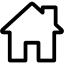## Gear profile

Under normal circumstances, involute gears are the way to go as they offer many advantages when compared to other gear profiles. However, if non-circular gears are required drawing an involute gear profile can become complicated as not all teeth are the same size.

As FDM 3d printed parts are (in general) not as strong as metal parts and therefore torque and transmission efficiency is not as important, we can try to use a different gear profile. If we use circular teeth we ensure that all teeth have the same size regardless of the gear outline.

This gear profile is therefore very easy to construct, as you can see in fig. 2 below.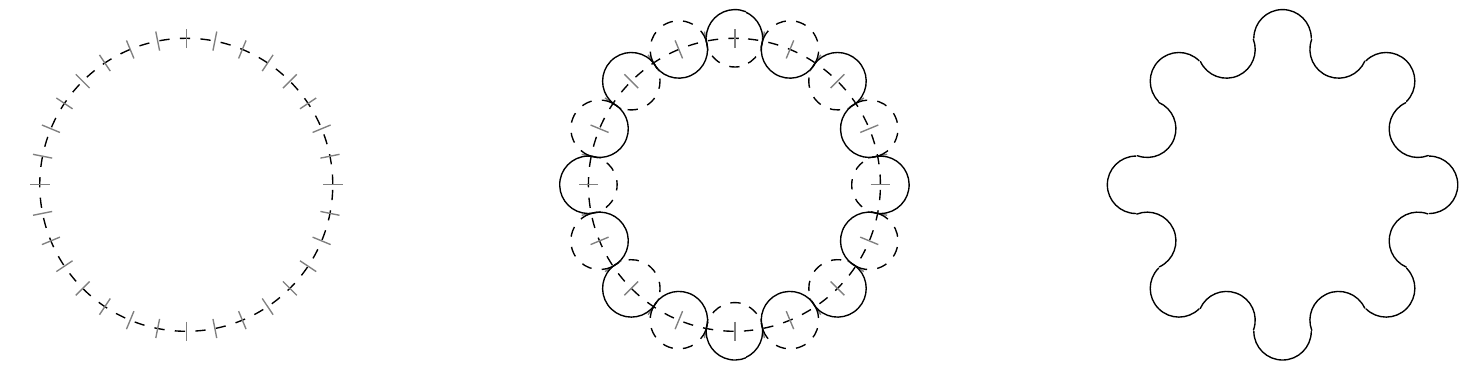Figure 2: How to construct a circular gear

## Calculating the correct tooth size

To calculate the required tooth size $$r$$ we have to solve a simple system of equations using a computer algebra program like maple for example.

As the required system of equations is dependant on the gear shape, please take a look at the linked PDF paper for more details on how to assemble it.

## Examples included in the PDF

The linked PDF paper contains several detailed examples of how to calculate both circular and non circular gears using the proposed gear profile. Listed below are the results of all included examples:

### Circular gears with given gear ratio and centerdistance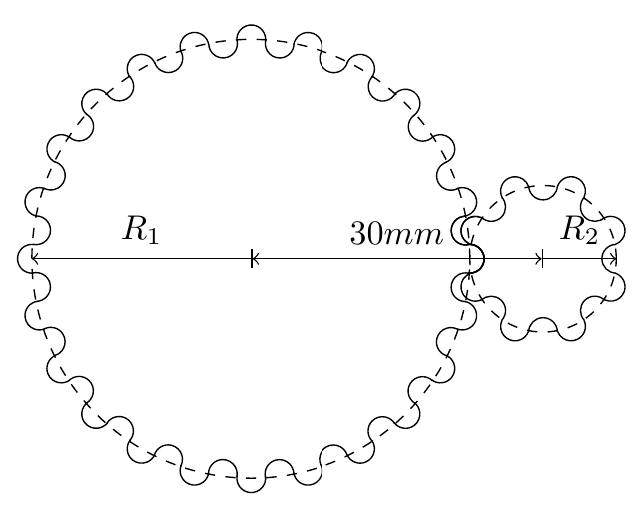Figure 3: Circular gears with given gear ratio and centerdistance

### Planetary gearbox (epicyclic gear train)Figure 3: Planetary gearbox (epicyclic gear train)

### Rectangular and circular gears with given gear ratio and center-distance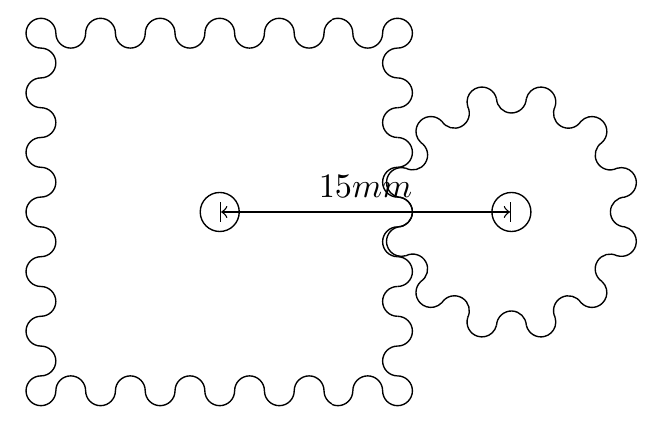Figure 6: Rectangular and circular gears with given gear ratio and center-distance

### Elliptical gear and square gear that fit together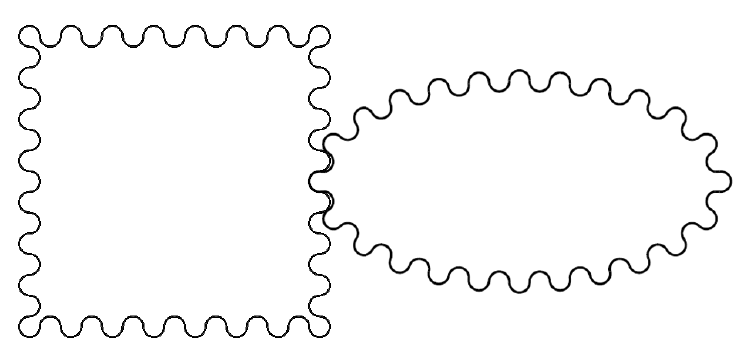Figure 8: Elliptical gear and square gear that fit together

## Get Notified of New Articles

Subscribe to get notified about new projects. Our Privacy Policy applies.